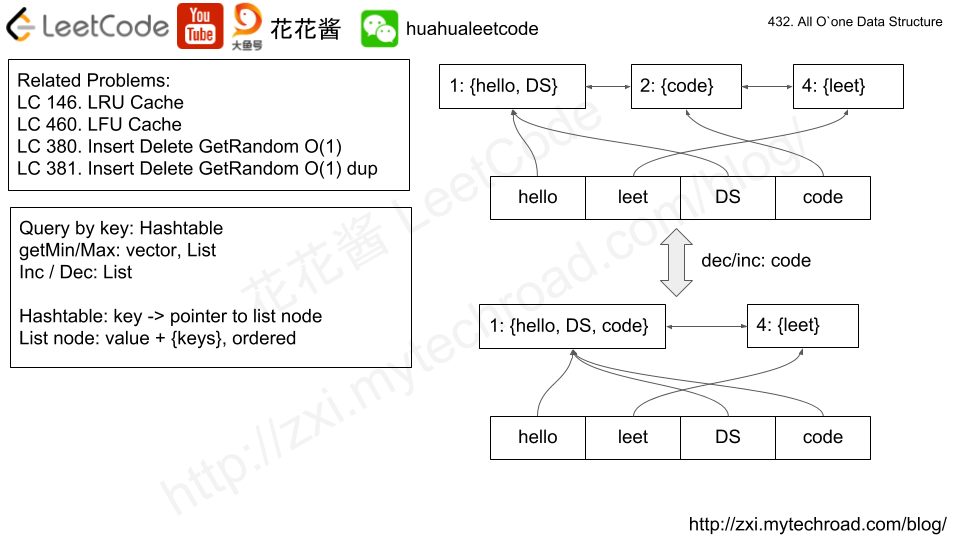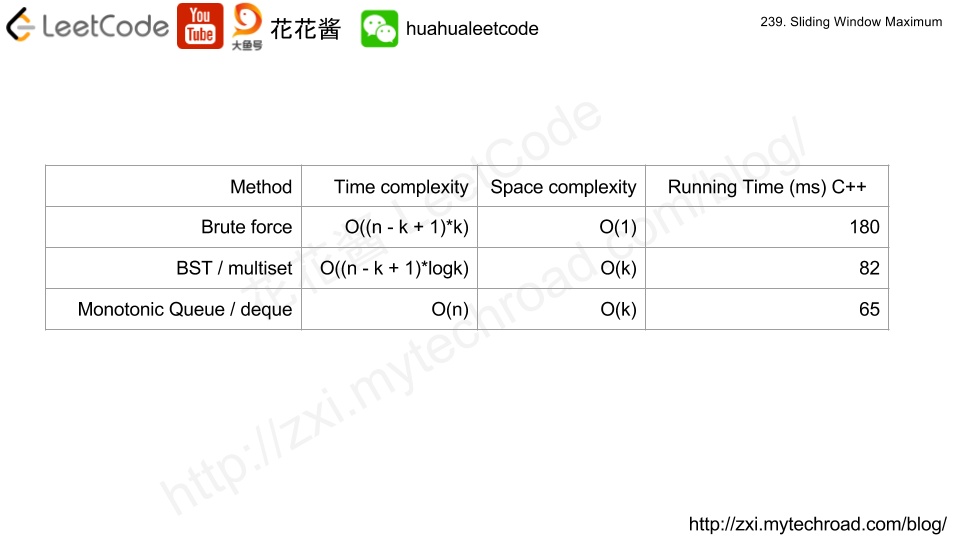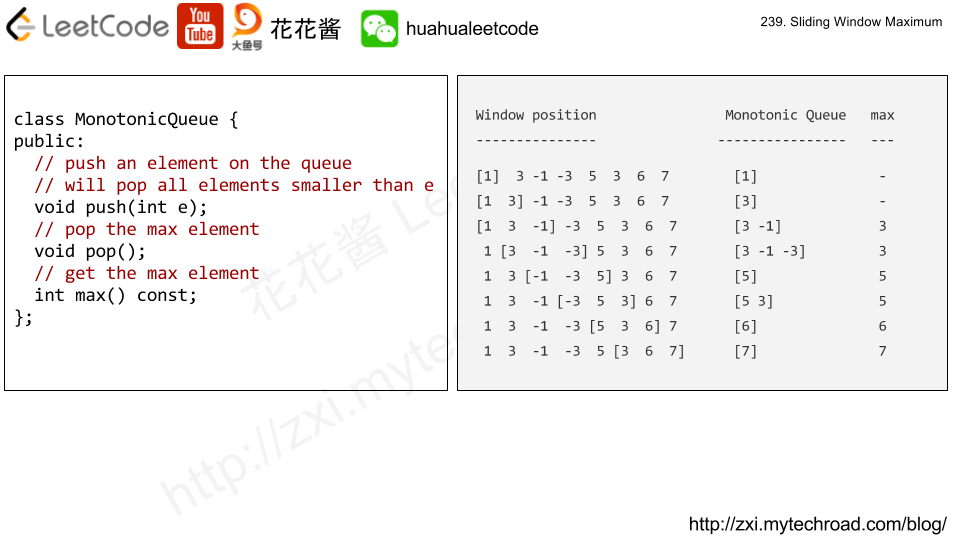# Posts published in “Data Structure”

Implement a SnapshotArray that supports the following interface:

• SnapshotArray(int length) initializes an array-like data structure with the given length.  Initially, each element equals 0.
• void set(index, val) sets the element at the given index to be equal to val.
• int snap() takes a snapshot of the array and returns the snap_id: the total number of times we called snap() minus 1.
• int get(index, snap_id) returns the value at the given index, at the time we took the snapshot with the given snap_id

Example 1:

Input: ["SnapshotArray","set","snap","set","get"]
[,[0,5],[],[0,6],[0,0]]
Output: [null,null,0,null,5]
Explanation:
SnapshotArray snapshotArr = new SnapshotArray(3); // set the length to be 3
snapshotArr.set(0,5);  // Set array = 5
snapshotArr.snap();  // Take a snapshot, return snap_id = 0
snapshotArr.set(0,6);
snapshotArr.get(0,0);  // Get the value of array with snap_id = 0, return 5

Constraints:

• 1 <= length <= 50000
• At most 50000 calls will be made to setsnap, and get.
• 0 <= index < length
• 0 <= snap_id < (the total number of times we call snap())
• 0 <= val <= 10^9

## Solution: map + upper_bound

Use a vector to store maps, one map per element.
The map stores {snap_id -> val}, use upper_bound to find the first version > snap_id and use previous version’s value.

Time complexity:
Set: O(log|snap_id|)
Get: O(log|snap_id|)
Snap: O(1)
Space complexity: O(length + set_calls)

# Problem

Design your implementation of the circular double-ended queue (deque).
Your implementation should support following operations:

• MyCircularDeque(k): Constructor, set the size of the deque to be k.
• insertFront(): Adds an item at the front of Deque. Return true if the operation is successful.
• insertLast(): Adds an item at the rear of Deque. Return true if the operation is successful.
• deleteFront(): Deletes an item from the front of Deque. Return true if the operation is successful.
• deleteLast(): Deletes an item from the rear of Deque. Return true if the operation is successful.
• getFront(): Gets the front item from the Deque. If the deque is empty, return -1.
• getRear(): Gets the last item from Deque. If the deque is empty, return -1.
• isEmpty(): Checks whether Deque is empty or not.
• isFull(): Checks whether Deque is full or not.

Example:

MyCircularDeque circularDeque = new MycircularDeque(3); // set the size to be 3
circularDeque.insertLast(1);			// return true
circularDeque.insertLast(2);			// return true
circularDeque.insertFront(3);			// return true
circularDeque.insertFront(4);			// return false, the queue is full
circularDeque.getRear();  				// return 32
circularDeque.isFull();				// return true
circularDeque.deleteLast();			// return true
circularDeque.insertFront(4);			// return true
circularDeque.getFront();				// return 4


Note:

• All values will be in the range of [1, 1000].
• The number of operations will be in the range of [1, 1000].
• Please do not use the built-in Deque library.

# Solution

Using head and tail to pointer to the head and the tail in the circular buffer.

# Problem

https://leetcode.com/problems/all-oone-data-structure/description/

Implement a data structure supporting the following operations:

1. Inc(Key) – Inserts a new key with value 1. Or increments an existing key by 1. Key is guaranteed to be a non-empty string.
2. Dec(Key) – If Key’s value is 1, remove it from the data structure. Otherwise decrements an existing key by 1. If the key does not exist, this function does nothing. Key is guaranteed to be a non-empty string.
3. GetMaxKey() – Returns one of the keys with maximal value. If no element exists, return an empty string "".
4. GetMinKey() – Returns one of the keys with minimal value. If no element exists, return an empty string "".

Challenge: Perform all these in O(1) time complexity.# Solution

Time complexity: O(1)

Space complexity: O(n), n = # of unique keys

# Related Problems

Problem:

https://leetcode.com/problems/implement-stack-using-queues/description/

Implement the following operations of a stack using queues.

• push(x) — Push element x onto stack.
• pop() — Removes the element on top of the stack.
• top() — Get the top element.
• empty() — Return whether the stack is empty.

Notes:

• You must use only standard operations of a queue — which means only push to backpeek/pop from frontsize, and is empty operations are valid.
• Depending on your language, queue may not be supported natively. You may simulate a queue by using a list or deque (double-ended queue), as long as you use only standard operations of a queue.
• You may assume that all operations are valid (for example, no pop or top operations will be called on an empty stack).

Idea:

Using a single queue, for every push, shift the queue (n – 1) times such that the last element becomes the first element in the queue.

e.g.

push(1): q: 

push(2): q: [1, 2] -> [2, 1]

push(3): q: [2, 1, 3] -> [1, 3, 2] -> [3, 2, 1]

push(4): q: [3, 2, 1, 4] -> [2, 1, 4, 3] -> [1, 4, 3, 2] -> [4, 3, 2, 1]

Solution:

Time complexity:

Push: O(n)

Pop/top/empty: O(1)

Space complexity: O(n)

C++

Problem:

https://leetcode.com/problems/sliding-window-maximum/

Given an array nums, there is a sliding window of size k which is moving from the very left of the array to the very right. You can only see the k numbers in the window. Each time the sliding window moves right by one position.

For example,
Given nums = [1,3,-1,-3,5,3,6,7], and k = 3.

Therefore, return the max sliding window as [3,3,5,5,6,7].

Note:
You may assume k is always valid, ie: 1 ≤ k ≤ input array’s size for non-empty array.

Could you solve it in linear time?

Idea:# Solution 1: Brute Force

Time complexity: O((n – k + 1) * k)

Space complexity: O(1)

# Solution 2: BST

Time complexity: O((n – k + 1) * logk)

Space complexity: O(k)

# Solution 3: Monotonic Queue

Time complexity: O(n)

Space complexity: O(k)

## Python3 V2

Mission News Theme by Compete Themes.